Chapter 9, Problem 9.117QPGeneral Chemistry - Standalone boo...

11th Edition
Steven D. Gammon + 7 others
ISBN: 9781305580343

Solutions

Chapter
SectionGeneral Chemistry - Standalone boo...

11th Edition
Steven D. Gammon + 7 others
ISBN: 9781305580343
Textbook Problem

Which of the following Lewis symbols is (or are) not correct? a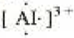b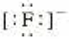c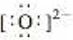d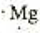e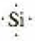Interpretation Introduction

Interpretation:

The incorrect Lewis symbols from the given set has to be identified.

Concept introduction:

Lewis structure, otherwise known as Lewis dot diagrams or electron dot structures that show the bond between atoms and lone pairs of electrons that are present in the molecule.  Lewis structure represents each atom and their position in structure using the chemical symbol.  Excess electrons forms the lone pair are given by pair of dots, and are located next to the atom.

Explanation

Reasons for incorrect options:

The given Lewis symbols of Al3+ ion is incorrect due to Al3+ ion contain three outer most electrons

Still sussing out bartleby?

Check out a sample textbook solution.

See a sample solution

The Solution to Your Study Problems

Bartleby provides explanations to thousands of textbook problems written by our experts, many with advanced degrees!

Get Started

Find more solutions based on key concepts